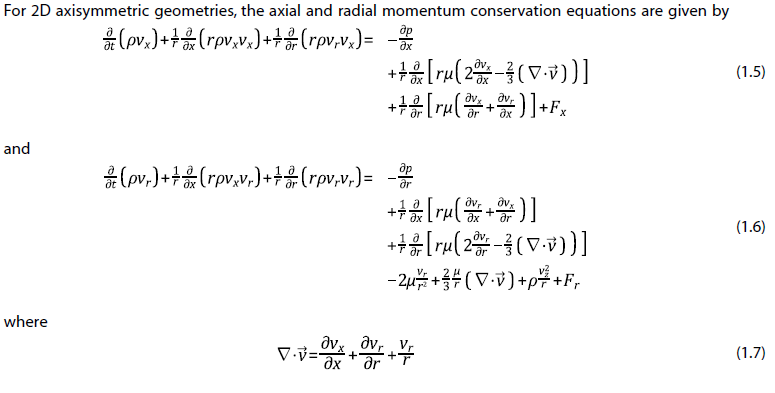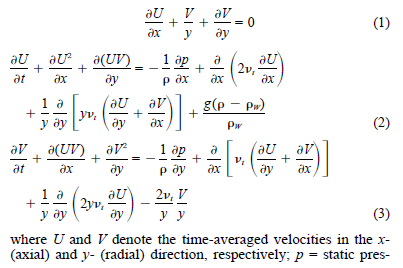# 网格讲师

### 成员列表

 下 下里巴人Alex
• RE: 欧拉双流体模型中应力张量的表示问题

@东岳 老师，没理解您的意思。后面的$\alpha_q\lambda_q\nabla·\vec{v_q}I$中单位二阶张量是表示体形变率仅出现在法相应力中，红框内等于$-\frac{2}{3}\lambda_q$时才能与法相应力对应啊

发布在 Algorithm
• RE: 二维轴对称动量方程

谢谢老师，我找出来看一下

发布在 Algorithm
• 二维轴对称动量方程上图是fluent theory guide中二位轴对称下的动量方程，下面是一篇文献中的二维抽对称动量方程。但对比文档中的方程，感觉文献里轴向的动量方程等式左边少一项$\frac{UV}{y}$,径向的方程等式左边少一项$\frac{VV}{y}$。
不知道问题出在哪里了，不知道有没有朋友能给点提示或者提供点有关二维轴对称方程的资料...发布在 Algorithm
• RE: 二维轴对称鼓泡塔稳态模拟不收敛

@东岳 这是瞬态模拟的气含率云图，此时的进出口流量接近平衡我尝试使用fluent稳态耦合求解器中的伪瞬态求解（Pseudo transient），获得了一个稳态收敛解，但是气含率和瞬态求解的结果相比偏小。

发布在 ANSYS Fluent
• RE: 掺混了纳米颗粒的煤油在圆管内的流动

可不可以把煤油和纳米粒子当做一个浆相，实验测定出浆相的物性，然后对这一相进行模拟？

发布在 ANSYS Fluent
• RE: 二维轴对称鼓泡塔稳态模拟不收敛

减小库朗数不应该更难收敛吗？我看文档上说减小库朗数可以使求解更稳定啊，增加库朗数可以加快收敛。
显示500步之后残差曲线也不下降，一直在某个范围波动。
在想是不是鼓泡塔就没有稳态，所以没法用稳态算

发布在 ANSYS Fluent
• 欧拉双流体模型中应力张量的表示问题

在Fluent theory guide中看到动量方程中应力张量表示如下图
红框中为什么是$\lambda_q-\frac{2}{3}\mu_q$?斯托克斯假设$\lambda_q=\frac{2}{3}\mu_q$，这里应该写$\lambda_q$或者$\frac{2}{3}\mu_q$啊，为什么这么表示？是我漏掉了什么吗？发布在 Algorithm
• 二维轴对称鼓泡塔稳态模拟不收敛

想模拟一个二维轴对称鼓泡塔，采用瞬态模拟时没有问题，但是模拟的时间比较长，看到文献中有采用稳态模拟的，设置后运行发现残差不下降，进出口流量也相差很大。
我的设置为：

1. 模型：二维轴对称，Standard k-epsilon dispersed，欧拉多相流模型，PBM（Discrete）
2. 边界条件：
• velocity inlet：$u_{l,in}=2.081m/s$，$k_{l,in}=0.0172$，$\epsilon_{l,in}=0.00287$，$u_{g,in}=2.081m/s$，入口气体体积分数为0.876
• pressure-outlet：出口处压力为21MPa
• Axi
1. 采用压力基Coupled求解器
2. 空间离散方式均为一阶
URF: Courant Number 50, Momentum 0.4. Pressure 0.5, Density 1, Body Force 1, Volume Fraction 0.3, k 0.8, e 0.8, Turbulent Viscosity 0.8, air bins 0.3
3. 初始化： k=0.005, e=0.005
希望有相关经验的朋友能给点建议发布在 ANSYS Fluent
• Fluent周期网格模拟有什么限制吗？

对一个带对称内构件的鼓泡塔进行模拟，是否可以采用周期网格模拟六分之一的模型呢？
这样模拟有什么限制吗，对周期边界处需要特殊处理吗？

发布在 ANSYS Fluent
• RE: 【请教】有关fluent在双路机器上运行的问题

是不是开着超线程？进BIOS里把超线程关闭试试

发布在 ANSYS Fluent